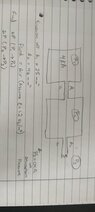# Pressure drop through volumes of compressible fluid

Natalinop
Homework Statement:
Pressure drop through volumes of compressible fluid
Relevant Equations:
deltaP = 1/2 (density)velocity^2)
I'm trying to estimate the pressure in the cavity, i.e. P2. I know the areas, A1 which in practicality is an annulus and A2 which is a 600m pipe with i/d 8mm.
I would like to initially understand how to calculate the pressure drop across the annulus.

At the moment I'm assuming the pressure drop which is not useful...
ΔP (P1-P2) = 2 kPa
v1 = SQRT(2000*2/1.2)= 57 m/s

Q1 (flowrate) = v1 * 26 = 1482 m2.mm/s

v2 = Q1/A2 = 1482/46 = 32 m/s

Friction losses at exit pipe
Fluid exits container at pipe length of 600mm length (L) and 8mm inner diameter (d)
L/d = 75
f = 0.05

ΔP (P2-P3) = 0.5*1.2*322*75*0.05= 2.3 kPa
NOt quiet sure how to calculate losses at entry and exit of pipe.

#### Attachments

•IMG20220522182506.jpg
19.3 KB · Views: 27